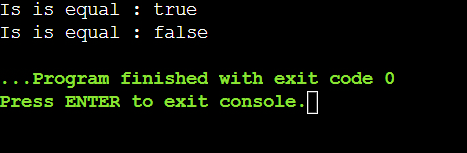Open in App
Not now

# Java File Class equals() Method with Examples

• Last Updated : 02 Feb, 2022

The equals() method of Java File Class compares the pathname supplied in the argument to the pathname provided in the argument. If the parameter is not null and points to the same file or directory, this function returns true. The operating system determines if the two abstract pathnames are equivalent or not.

Syntax:

`public boolean equals(Object obj)`

Parameters:

• obj – The item against which the abstract pathname is to be compared

Return Value: It returns true, if and only if the items are the same; otherwise, false.

Example:

## Java

 `// Java program to show the usage of  ``// File Class equals() Method``import` `java.io.File;`` ` `public` `class` `Main {``    ``public` `static` `void` `main(String[] args)``    ``{``        ``boolean` `bool;``        ``try` `{`` ` `            ``// create new files``            ``File f1 = ``new` `File(``"Gfg.txt"``);``            ``File f2 = f1;``            ``File f3 = ``new` `File(``"Gfg2.txt"``);`` ` `            ``// returns boolean value``            ``bool = f1.equals(f2);`` ` `            ``// prints the output``            ``System.out.println(``"Is is equal : "` `+ bool);`` ` `            ``// returns boolean value``            ``bool = f1.equals(f3);`` ` `            ``// prints the output``            ``System.out.print(``"Is is equal : "` `+ bool);``        ``}``        ``catch` `(Exception e) {``            ``// if any error occurs``            ``e.printStackTrace();``        ``}``    ``}``}`

The method compares two File instances to see whether they are the same. This approach does not compare the content of the file or directory; instead, it checks if the pathnames are the same.

Output:My Personal Notes arrow_drop_up